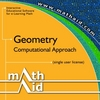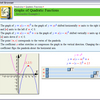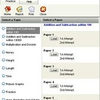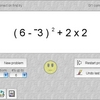Math practice

Math Practice is an easy to use software addressed to parents who wish to help kids make their first steps into the world of math, providing fairly simple teaching tools and a basic interface. Choose between addition, subtraction, multiplication and division. Once you click on one of these, math exercises are automatically launched, pick difficulty by choosing between three different levels. Math Practice does work flawlessly on all Windows versions and runs on low resources all the time, which makes it appropriate for all types of adult users who wish to teach children the basics of math.

Related software (5)MathAid Geometry

Tutorial contains basic concepts, interactive examples, and problems with randomly generated parameters. A customer is allowed to select chapters for a test, get the test review, ...MathAid Precalculus

Tutorial contains basic concepts, interactive examples, and problems with randomly generated parameters. A customer is allowed to select chapters for a test, get the test review, ...Mathematics Quiz

For students aged 7 - 9 in Primary 1 - 3. There are over 1500 challenging Maths quizzes and problem sums to practise on. Topics include Addition, Subtraction, Multiplication, Divi ...Smooth Operators

Smooth Operators is a complete solution for learning, practicing, and testing the order of operations. An interactive lesson teaches concepts. Practice by clicking operands, or tes ...Math Practice

Math Practice caters for everyone. Get to grips with math ranging from basic addition and subtraction to working with exponents. Questions are automatically generated and then mark ...